Structure-aware Fisheye Views for Efficient Large Graph Exploration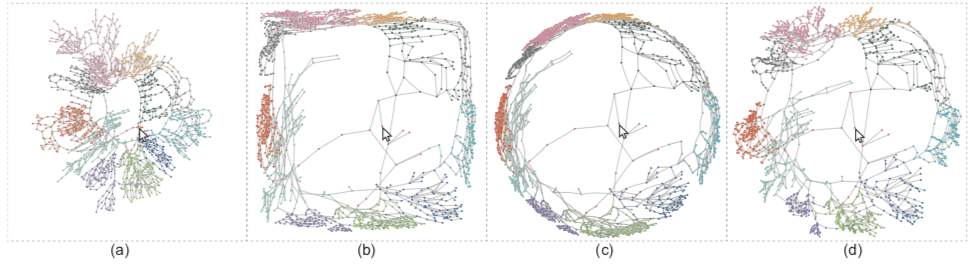• 论文原标题： Structure-aware Fisheye Views for Efficient Large Graph Exploration

一、介绍

1. 最小化结构的扭曲；
2. 提升感兴趣区域的可读性；
3. 在交互的时候要维护连续性。

1. 多焦点透镜：在多个焦点附近高亮结构
2. 聚类透镜：可以维持一个子结构
3. 路径透镜：可以聚焦到一个用户指定的路径上

1. 提出了一个统一的框架，能够生成用于探索大图的结构相关的鱼眼视图。
2. 设计了一组任务导向的鱼眼透镜，适用于不同的可视化任务。
3. 利用基于GPU的方法进行实现，并进行了一次评估，一个user study，以及两个case study。

二、相关工作

图探索技术

pan-and-zoom，fisheye views，semantic zooming，dynamic layout

高质量图布局技术

stress-based methods：将几何限制转换成优化问题。早期的工作专注于方向的限制。但是这个优化问题往往是NP问题。Dig-CoLa方法则有效的解决了有限制的布局的优化问题，通过将限制集成为压力优化（stress majorization）。

三、背景

几何鱼眼

graphical fisheye，hyperbolic fisheye，iSphere

Edge Vector-based Stress Majorization（布局方面）

$$arg\min_X\sum_{i<j}w_{ij}(x_i-x_j-e_{ij}d_{ij})^2$$

e为边的目标方向，d为边的目标长度。在这种布局方法的启发下，作者期望通过改变边的朝向跟长度，来产生一个结构相关的并且能够光滑过渡的鱼眼交互。

四、结构相关的鱼眼（structure-aware fisheye）

$$arg\min_{Z^t}\sum_{(i, j) \in E} \omega_{ij}^s||z_i^t-z_j^t-e_{ij}^sd_{ij}^s||^2 \\ +\sum_{(i, j) \in \Omega} \omega_{ij}^r||z_i^t-z_j^t-e_{ij}^rd_{ij}^r||^2 \\ +\sum_{i \in \lbrace 1\ldots n \rbrace} \omega_i^t||z_i^t-z_i^{t-1}||^2 \\$$

结构相关的限制

$$e_{ij}^s=\frac{x_i-x_j}{||x_i-x_j||}, d_{ij}^s=||x’_i-x’_j||$$

$X’$表示的是用鱼眼透镜估计的目标位置。保持了方向的一致性。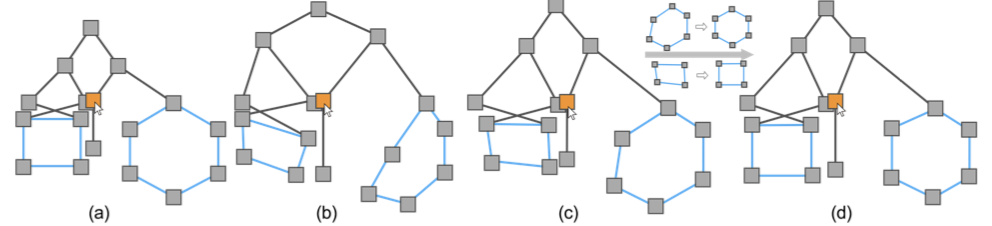$$\min_{\rho} \sum_{(i, j) \in E_s}(\rho d_{ij}-d’_{ij})^2$$

$$d_{ij}^s=\rho d_{ij}$$

可读性限制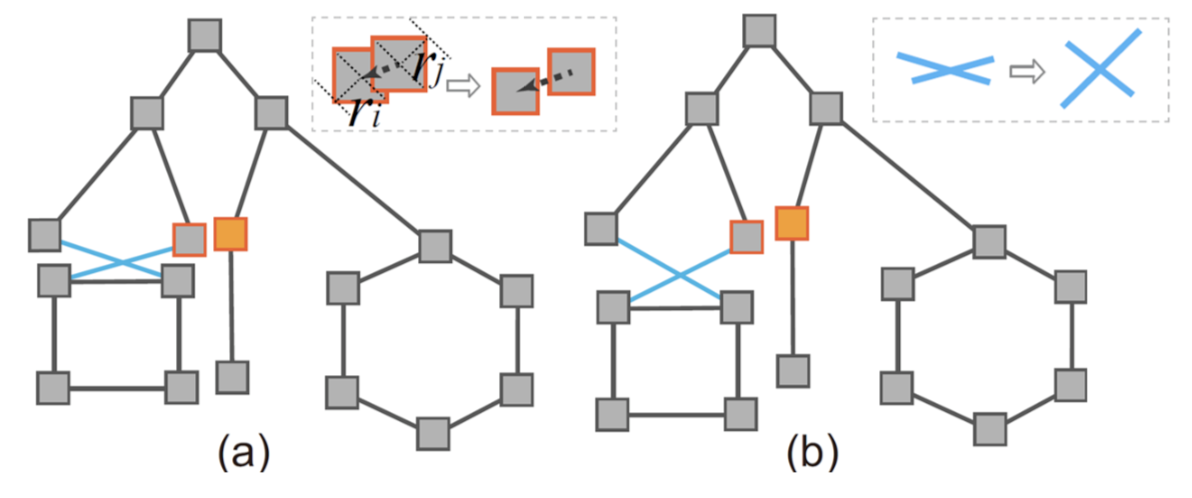$$d_{ij}^r=r_i+r_j+\varepsilon, e_{ij}^r=e_{ij}^s$$
$r_i$和$r_j$是节点包围盒的半径，$\varepsilon$则是节点分离系数（设为屏幕尺寸的1%）。当然这中间还包括了那些不出现的虚拟边（也就是没有相连的两个节点之间也要分离）

Purchase的可读性研究证明，边的相交很能影响可读性。完全解决边相交的问题不可能，特别是大图。最近的研究表明最大化夹角能够提升路径追踪任务的能力。
$$e_{ij}^r=e_{ij}^s\oplus\frac{\pi - \alpha}{2}, e_{lk}^r=e_{lk}^s\ominus\frac{\pi - \alpha}{2}$$

五、任务导向的鱼眼透镜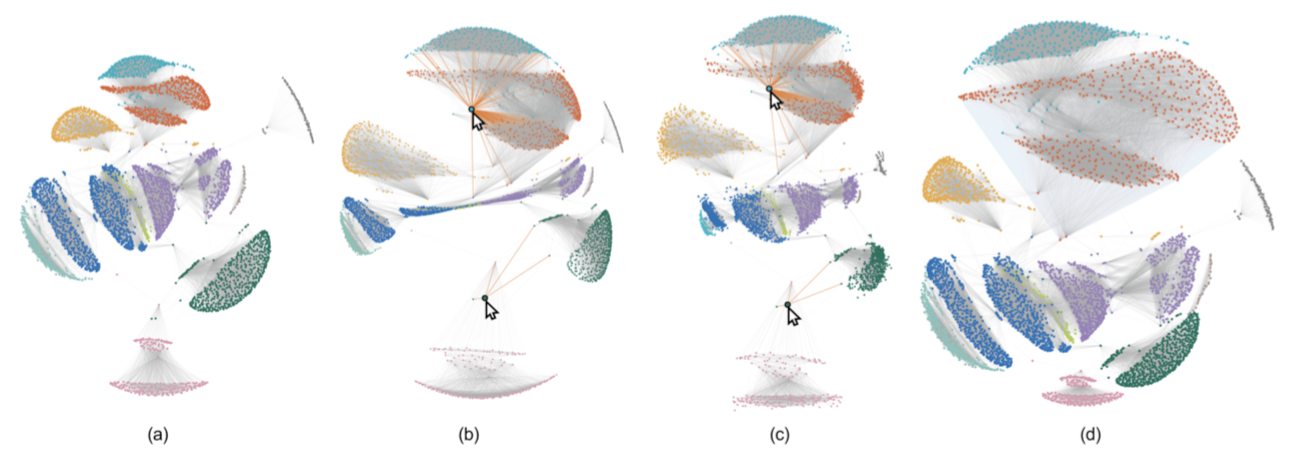六、结果和评估

定量比较

• graphical fisheye(GF, M. Sarkar and M. H. Brown. Graphical fisheye views of graphs. In Proc. SIGCHI conference on Human Factors in Computing Systems, pp. 83–91, 1992. doi: 10.1145/142750.142763)

• hyperbolic fisheye(HF, T. Munzner. Exploring large graphs in 3d hyperbolic space. IEEE Computer Graphics and Applications, 18(4):18–23, 1998. doi: 10.1109/38.689657)

• iSphere(F. Du, N. Cao, Y.-R. Lin, P. Xu, and H. Tong. isphere: Focus+ context sphere visualization for interactive large graph exploration. In Proc.SIGCHI conference on Human Factors in Computing Systems, pp. 2916–2927, 2017. doi: 10.1145/3025453.3025628)

• 边方向保留
• 节点重叠
• 形状保留

$$EOO(Z)=1-\frac{1}{|E|}\sum_{(i,j) \in E}||\langle \frac{x_i-x_j}{||x_i-x_j||}, \frac{z_i-z_j}{||z_i-z_j||} \rangle||$$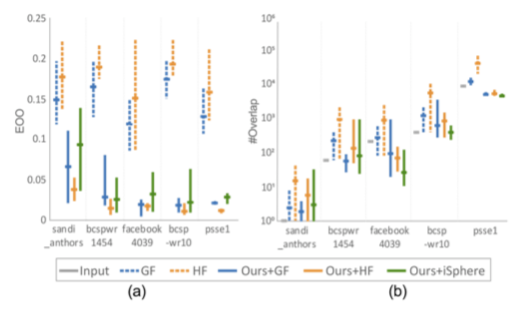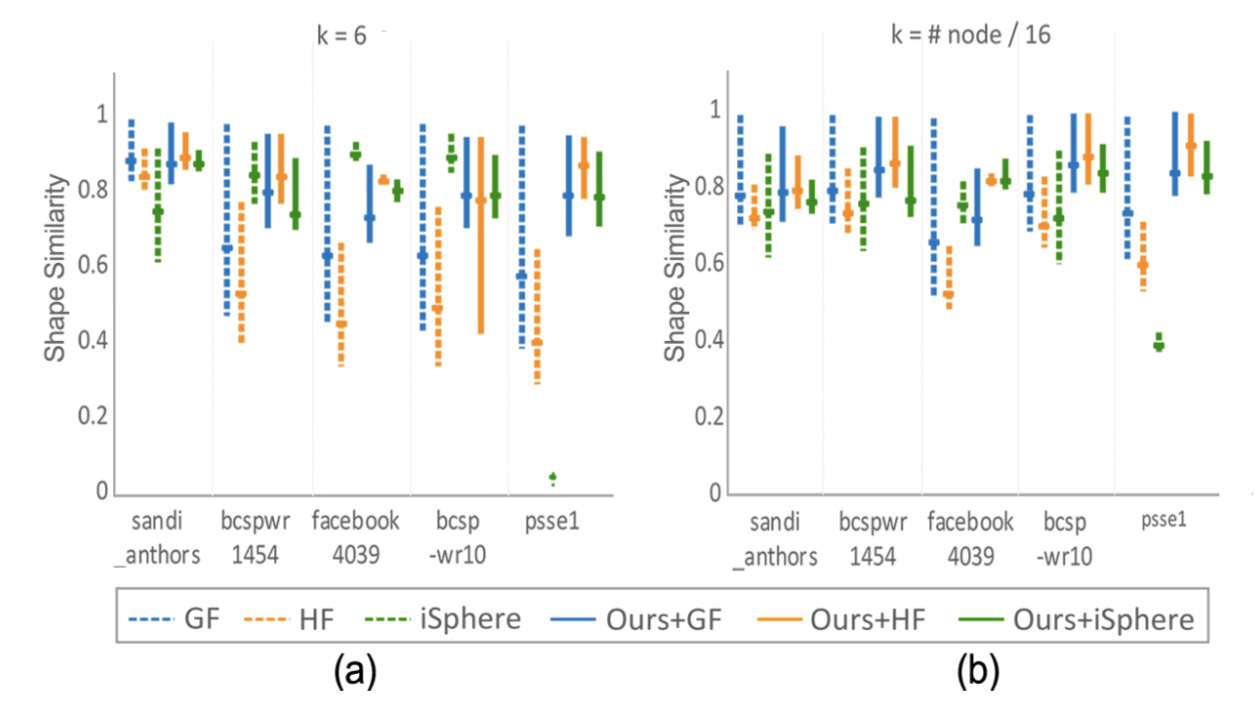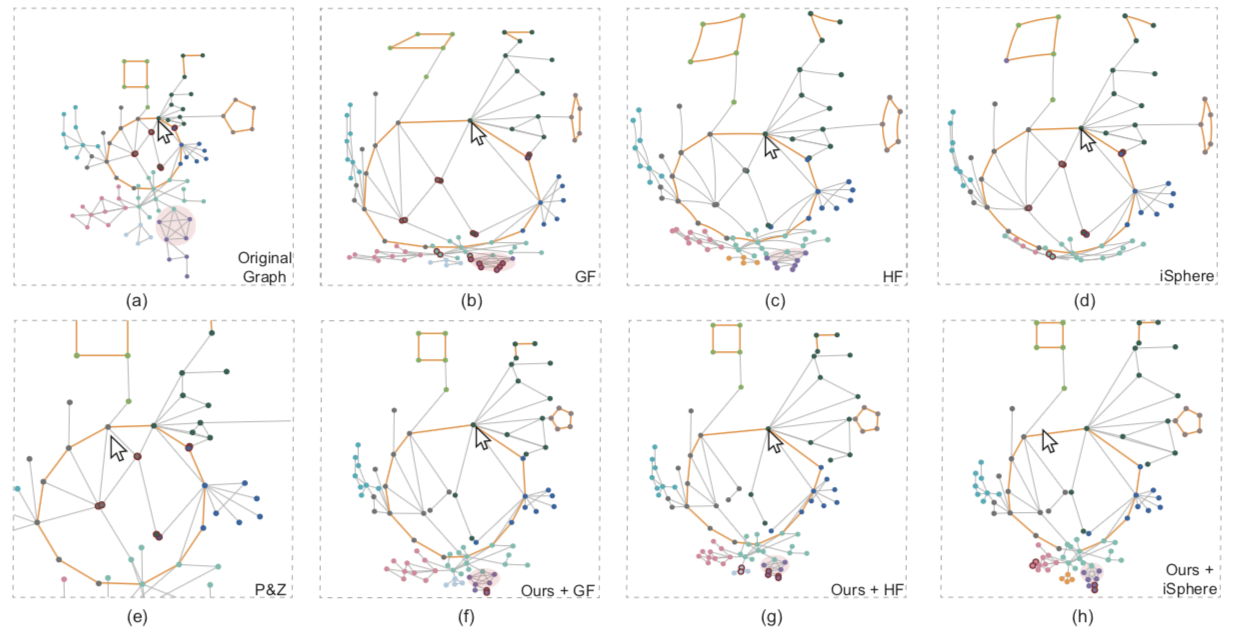Lab Stydy

• pan-and-zoom(P&Z)
• graphical fisheye(GF)
• hyperbolic fisheye(HF)
• iSphere
• 文章方法+graphical fisheye(Ours+GF)，因为这个表现适中，没有很好也不是很坏。

实验条件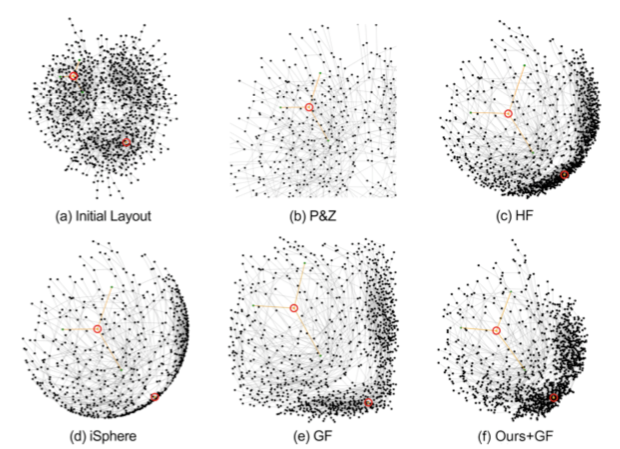1. 用直线渲染的技术（GF, P&Z, Ours+GF）会比曲线（HF，iSphere）来的高效。
2. 鱼眼技术（GF，HF，iSphere和Ours+GF）则会比没有context的技术（P&Z）更高效。
3. 低畸变率（Ours+GF，P&Z）会比高畸变率（GF，HF，iSphere）更高效。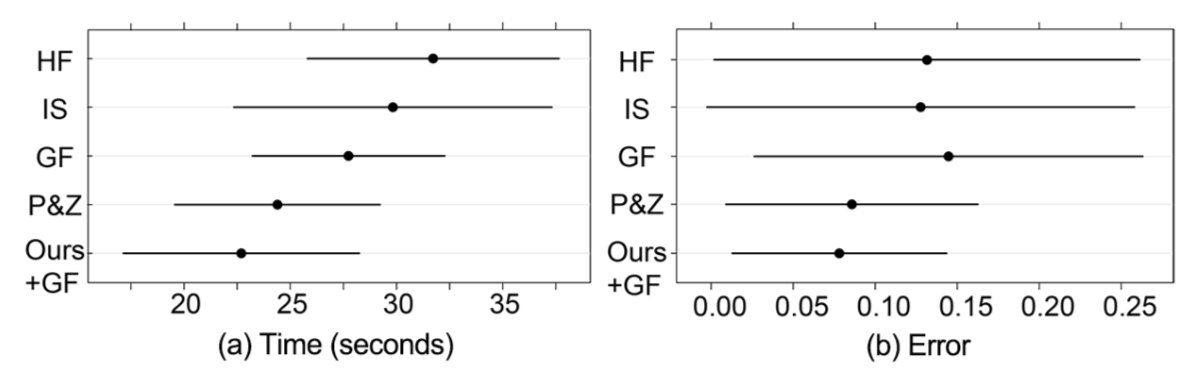讨论

Hyperbolic vs. Pan&Zoom：被试提到：“双曲线鱼眼会导致挤压跟变形，有些边甚至会有很大的曲率，而平移缩放则保持了一个稳定的布局以便进行路径探索，尽管丢失了一部分上下文”。

iSphere：虽然iSphere文章本身说明了该技术比其他的技术在路径探索任务上更好，但是本文却只显示了这个技术略微比HF要好，却比其他的技术要差。被试提到，“iShpere在放大的时候，容易丢失一些远离的节点”，因为iShpere只渲染了焦点区域内的节点。有一些被试则没有感知到iSpher带来的畸变，这让他们觉得iShpere的交互像是在旋转一个球体，就跟平移缩放一样。

Graphical fisheye：相比于HF和IS，GF用了直线边，因此更短时间就完成了任务，然而GF的畸变导致了整体结构的破坏，于是它的错误率是最高的。被试提到：“GF的交互不够直观，而且它的畸变也是相对无法理解”

Our fisheye：虽然文章的方法基于GF，然而却得到了最好的表现。被试提到：“这个结构相关的鱼眼结合了双曲线和缩放平移的优点，直线边则有助于进行路径探索，而且即便会比较小，并且它的交互会显得更快，因为点击一个节点会放大感兴趣全区域而不用进行缩放平移”。

案例研究

美国主要城市的探索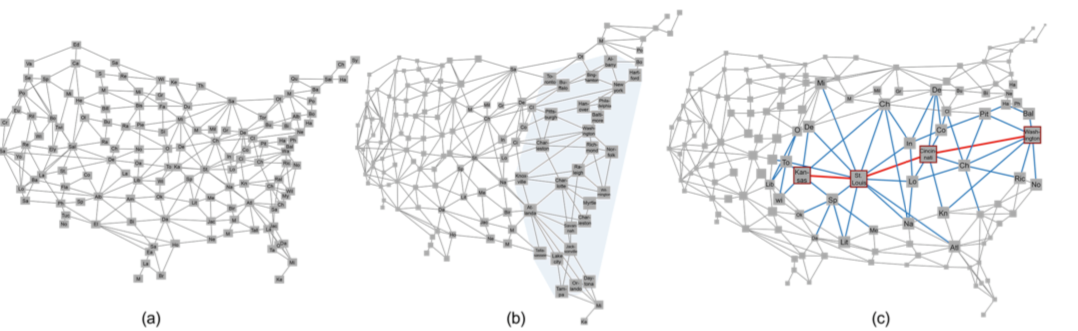134个节点代表美国城市，338边则表达了城市之间是相邻的关系。图b利用了聚类透镜，放大了淡蓝色区域。于是节点之间的重叠降低，可以看清这些节点的label。